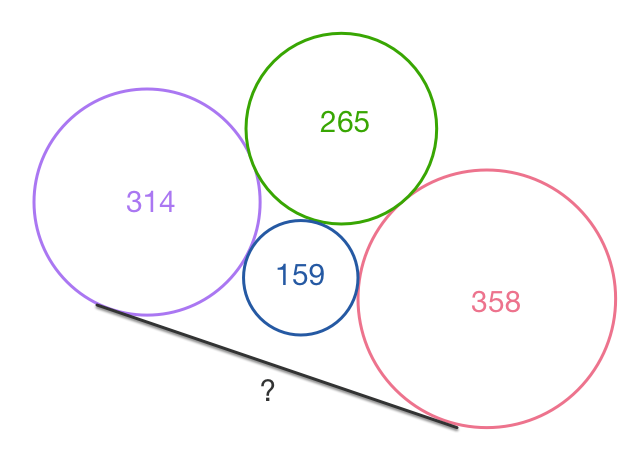# Pi Day Challenge 2016 - Adagio

Geometry Level 5The diagram shows two circles with diameters 314 and 358 which share a common tangent.

Both circles are tangent to the two smaller circles in the middle with diameters 265 and 159.

The length of the tangent in its simplest form can be expressed as $\dfrac{\sqrt{15}}{4}(a\sqrt{b}+\sqrt{c}),$ where $a,b$ and $c$ are positive integers with $b,c$ square-free. Find $a+b+c$.

###### Happy Pi Day 2016.

Index of Pi Day Challenges

×